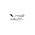Keli1K 1klan Ya Karna Itu Untuk Kemajuan Dan Rizki Admin Biar Nambah semangat Berbagi Materi

# Basic Laboratory Techniques ( Theory )

Chemistry is an experimental science. It depends upon careful observation and the use of good laboratory techniques. In this experiment you will become familiar with some basic operations that will help you throughout this course. Your success as well as your safety in future experiments will depend upon your mastering these fundamental operations.

Because every measurement made in the laboratory is really an approximation, it is important that the numbers you record reflect the accuracy of the device you use to make the measurement. Appendix A of this manual contains a section on significant figures and measurements that you may find helpful in performing this experiment. Our system of weights and measures , the metric system , is based mainly upon fundamental properties of one of the world's most abundant substances , water.

#### Laboratory Balance

A laboratory balance is used to obtain the mass of various objects. There are several different varieties of balances , with various limits on their accuracy. Five of these balance are figure in this picture below, Most modern laboratories posses single pan balances with only two knife edges. These are the most accurate balances ; generally , they are also the simplest to use and are the most delicate and expensive. A comparison of the basic operation of a single pan balance and a double pan balance.

Unit of measurement in the metric system

Mass or Weight = Gram ( g ) = weight of 1 cubic centimeter of water at 4 C and 760 mmHg
mass = quantity of material
weight = mass x gravitational force

Length = meter ( m ) = 100 cm = 1000 mm = 39,37 inch

Volume = Liter = Volume of 1 kilogram of H2O at 4 C

Temperature = Measures heat intensity  ( K )

Heat = Calorie , amount of heat required to raise 1 g of water 1 C, 1 cal = 4,184 joule

Density = d usually g/ml, d = mass / unit volume

Specific Gravity = sp gr, dimensionless

#### Bunsen Burner

The bunsen burner is convenient source of heat in the laboratory. Although there are several varietes, their principle of operation is the same and is similiar to that of the common gas stove. the bunsen burner requires gas and air ,Which it mixes in various proportions . The amount of air and gas mixed in the chamber is varied by use of the two adjustements.

#### Meter Rule

The standard unit of length is the meter , which is 39,37 in. in length A metric rule, or meter stick, is divided into centimeters ( 1 cm = 0,01 m ) and milimeters ( 1 mm = 0,001 ) , it follows that 1 in = 2,54 cm.

Graduated cylinder are tall, cylindrical vessels with graduations scribed along the side of the cylinder. Since volumes are measured in these cylinders by measuring the height of a column of liquid. it is critical that the cylinder have a uniform diameter along its entire height. Obviously, a tall cylinder with a small diameter will be more accurate than a short one with a large diameter. A liter is divided into milimeters such that 1 ml = 0,001 L, 1L = 1000 ml.

#### Thermometers

Most thermometers are based upon the principle that liquids expand when heated. Most common thermometers use mercury as liquid. These thermometers are constructed so that a uniform-diameter capillary tube surmounts a mercury reservoir. To calibrate a thermometer , one defines two reference points, normally the freezing point of water ( 0 C ) and the boiling point of water ( 100 C ) at 1 atm of pressure . Once these points are marked on the capillary , its length is then subdivided into uniform divsions called degrees . Obviously, there are 100 C between these two points on the centrigrade Celcius , C scale and 180 C between these two points on the Fahrenheit ( F ) scale.

#### Pipets

Pipets are glass vessels that are constructed and calibrated so as to deliver a precisely known volume of liquid at a given temperature. Always use a rubber bulb to fill a pipet , never use your mouth !

It is important that you be aware that every measuring device, regardless of what it may be, has limitations in its accuracy. Moreover, to take full advantage of a given measuring instrument you should be familiar with or evaluate its accuracy . Careful examination of the subdivisions on the device will indicate the maximum accuracy you can expect of that particular tool. In this experiment you will determine the accuracy of your 10 ml pipet. The approximate accuracy of some of the equipment you will use.

Not only should you obtain a measurement to the highest degree of accuracy that the device or instrument permits, but you should also record the reading or measurement in a manner that reflect the accuracy of the instrument. For example , a mass obtained from an analytical balance should be observed and recorded to the nearest 0,0001 g.

Equipment Accuracy Laboratory

Analytical balance = 0,1 mg

Meter Stick = 0,1 cm

Pipet = 0,02 ml

Buret = 0,02 ml

Thermometer = 0,2 C

### 2 Responses to "Basic Laboratory Techniques ( Theory )"

1.Kacau hambuih Sosoh.

2.Nice post! This is a very nice blog that I will definitively come back to more times this year! Thanks for informative post. medical equipment and supplies

Apakah Sobat Masih Bingung ? Jangan Malu-Malu Sampaikan Saja Keluh Kesah Sobat Di Kolom Komentar !